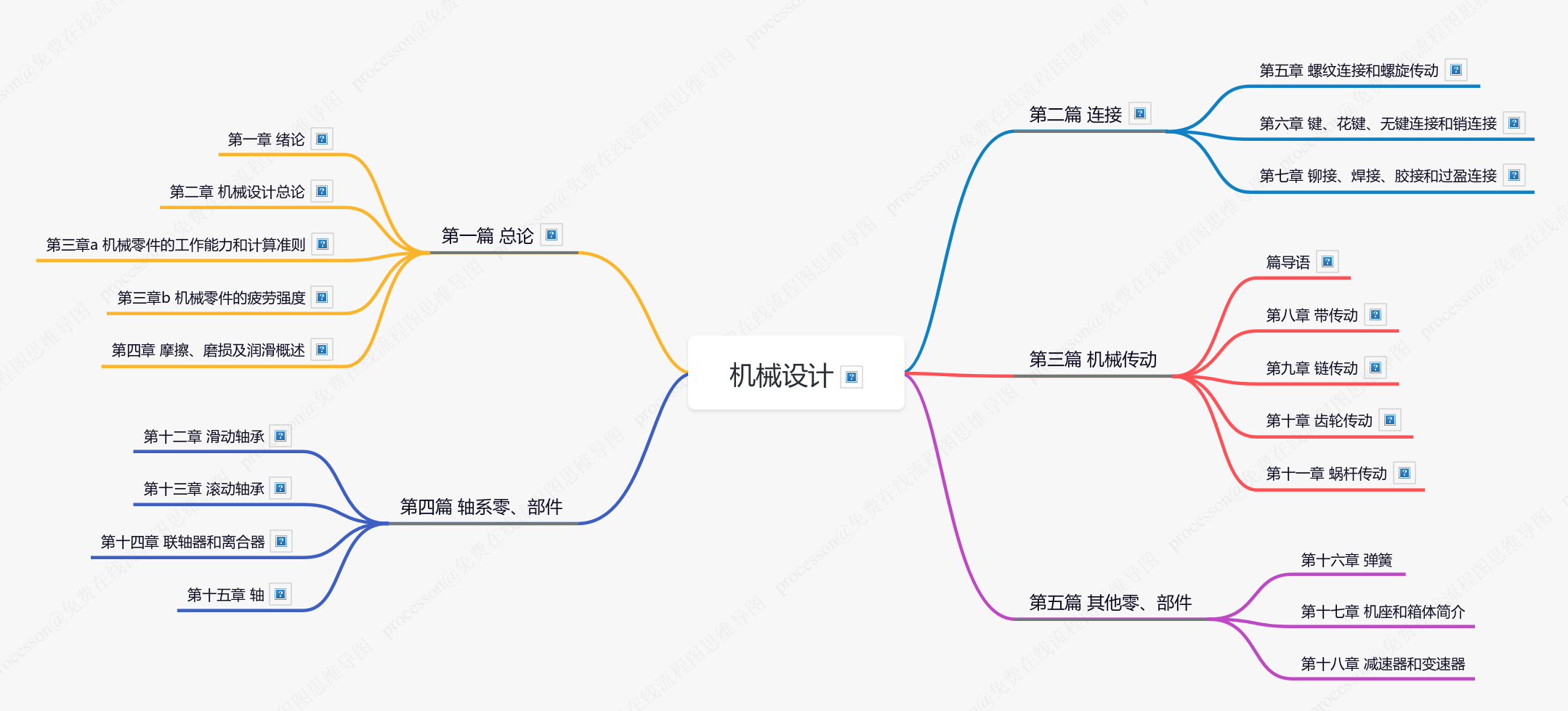# 首页

1. 1.
适合正在学习机械设计课程或准备相关专业课考研的同学
2. 2.
比原教材更“干”，条理更清晰（详见下方“展示段落”中的对比），便于快速且详尽地浏览所有知识点内容、构建图谱式的知识体系
3. 3.
以西北工业大学濮良贵版教材为主线，辅以邱宣怀版教材内容补充
4. 4.
5. 5.
加入更多注解、高清实物图、动图，便于建立认知、加深理解
6. 6.
几乎所有教材中提到的国家标准文件（GB(/T)文件）都可以直接下载最新版本，部分章节还额外附录更多相关国标文件
7. 7.
离线版（PDF 翻页阅读，排版精美）已经开始筹划，未来会在本页面发布下载链接，敬请期待

2021.7 ~ 2022.1

## 思维导图目录## 段落展示

4.链条的节距
$p$

$p$

$p$

• 总体尺寸增大
• 多边形效应显著
• 振动、冲击、噪声更严重

——

——

$P_0$
$P_0 = \frac{([\sigma]-\sigma_{b1}-\sigma_c)(1 - \frac{1}{e^{f_v \alpha}})A v}{1000}$

$P_0$

$[\sigma]$

$\sigma_{b1}$

$\sigma_c$

$f_v$

$\alpha$

 ​​ ​​ ​​ ​​

## 打赏与捐赠